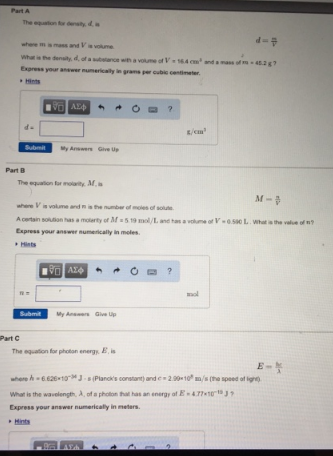# Problem: Part AThe equation for density, d is d = m/Vwhere m is mass and V is volume. What is the density, d, of a substance with a volume of V = 16.4 cm3 and a mass of m = 45.2 g? Express your answer numerically in grams per cubic centimeter.Part B The equation for molarity, M, is M = n/V where V is volume and n is the number of moles of solute.A certain solution has a molarity of M = 5.19 mol/L and has a volume of V = 0.590 L. What is the value of n? Express your answer numerically in moles. Part CThe equation for photon energy, E, is E = hc/λ where h = 6.626 x 10-34 J•s (Planck's constant) and c = 299 x 108 m/s (the speed of light).What is the wavelength, λ, of a photon that has an energy of E = 4.77 x 10-19 J? Express your answer numerically in meters.

###### FREE Expert Solution
96% (250 ratings)###### Problem Details

Part A

The equation for density, d is

d = m/V

where m is mass and V is volume.

What is the density, d, of a substance with a volume of V = 16.4 cm3 and a mass of m = 45.2 g?

Part B

The equation for molarity, M, is

M = n/V

where V is volume and n is the number of moles of solute.

A certain solution has a molarity of M = 5.19 mol/L and has a volume of V = 0.590 L. What is the value of n?

Part C

The equation for photon energy, E, is

E = hc/λ

where h = 6.626 x 10-34 J•s (Planck's constant) and c = 299 x 108 m/s (the speed of light).

What is the wavelength, λ, of a photon that has an energy of E = 4.77 x 10-19 J?